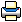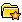Avner Magen (1968 - 2010)HomeBiography## Avner’s academic researchBy close colleagues of AvnerProf. Avner Magen was a brilliant scholar, making fundamental contributions to a number of areas of theoretical computer science that include metric embeddings, sublinear algorithms, convex programming, computational geometry and approximation algorithms.

Avner completed his undergraduate and graduate studies at the Hebrew University of Jerusalem, and received his Ph.D. in Computer Science in 2002, under the supervision of Prof. Nati Linial. He held a postdoctoral fellowship at NEC Research in Princeton, New Jersey, from 2000 until 2002. He joined the University of Toronto in 2002, first as a postdoctoral fellow, and then as an Assistant Professor in 2004. He was promoted to Associate Professor in 2009.

Avner was a wonderful colleague with a terrific sense of humor, great energy, and tremendous warmth. He was a dedicated research supervisor and a superb teacher whose mentorship inspired his students and those around him. He was one of theoretical computer science's most creative researchers. The loss of such mathematical talent will have a profound impact on his colleagues, students, and the entire research community.

Below we try to give a glimpse of some of Avner's fundamental scientific contributions.

Metric Embeddings
Avner's Ph.D. thesis was in the area of metric embeddings. A metric space is the standard way in which mathematicians investigate the notion of distance. You can think of a set of cities and their mutual distances, or you could consider the time it takes for a message to go between pairs of computers in a network. A metric can be very unwieldy and hard to analyze. On the other hand, there are certain metric spaces which are very nice and understandable. For example take a set of points in space and consider their (straight line) distances. A natural approach to the study of complicated metric spaces is this:

Try to approximate a given complicated metric space in terms of a "nice" metric space. To what extent can this be done? Such questions have been in the focus of intensive investigations over the past 15 years or so. They turn out to be of interest in the pure mathematical sense and in computational applications. This was the general area of Avner's thesis work. He studied such questions mostly for metrics that come from graphs. His work involves many ideas: combinatorial, algorithmic and geometric.

Sublinear Algorithms and Computational Geometry

Avner and co-authors made fundamental contributions to the field of sublinear algorithms. For example, he showed how to tell if two strings of characters are either nearly identical or far apart, while doing so in much less time than it would take to read the strings in the first place.

Avner's work in sublinear geometric algorithms pioneered a new theme of research that has since thrived. One of his major contributions addressed this question: given a polytope in Euclidean 3-space, is it possible to approximate the shortest distance between two points on its boundary by looking at only a tiny portion of the polytope?  In general, the answer is no. If the polytope is convex, however, then sampling its surface at random in a few places provides enough "geodesic" information to make shortest paths computation possible. In fact, by looking at as few as square root of the total number of vertices, Avner and co-authors showed that it is possible to approximate the shortest path length with arbitrarily small relative error. This counterintuitive result, which turns out to be optimal, rests on a sophisticated new construction of constant-size approximations of convex structures. The basic technique is so general it can be extended to provide sublinear algorithms for evaluating volumes, detecting intersections, shooting rays, performing point location in Voronoi diagrams, etc.

Among Avner's other contributions in this area was a beautiful algorithm for approximating the weight of the Euclidean minimum spanning tree in sublinear time.

Approximation algorithms
Avner's research in the last five years has focused on the limitations of prominent algorithmic techniques for hard combinatorial optimization problems. Optimization problems abound in practical applications but for many of them, no exact and efficient algorithms are known. To overcome this obstacle a common approach is to sacrifice optimality while insisting on efficiency. The question raised is, what are the approximation guarantees for algorithms for solving important optimization problems, given limited computational resources? Apart from its strong practical importance, this question touches on fundamental and deep mathematical questions that have remained unresolved for decades. In his work, Avner has established strong lower bounds for the approximability for many combinatorial optimization problems for algorithms based on convex programming, an algorithmic technique which is central in the theory of approximation algorithms. Similar hardness results have been rare in complexity theory and they are essential for practical applications as they reveal the weaknesses of the most prominent known algorithmic paradigms.

An example of Avner's genius in this area is a tight integrality gap for the vertex cover problem. He and coauthors proved essentially that a huge class of semidefinite programming algorithms for the famous vertex cover problem will not achieve a solution of value less than the value of the optimal solution times a factor of two.

Here is a list of Avner Magen's publications. http://www.cs.toronto.edu/~avner/pub.html